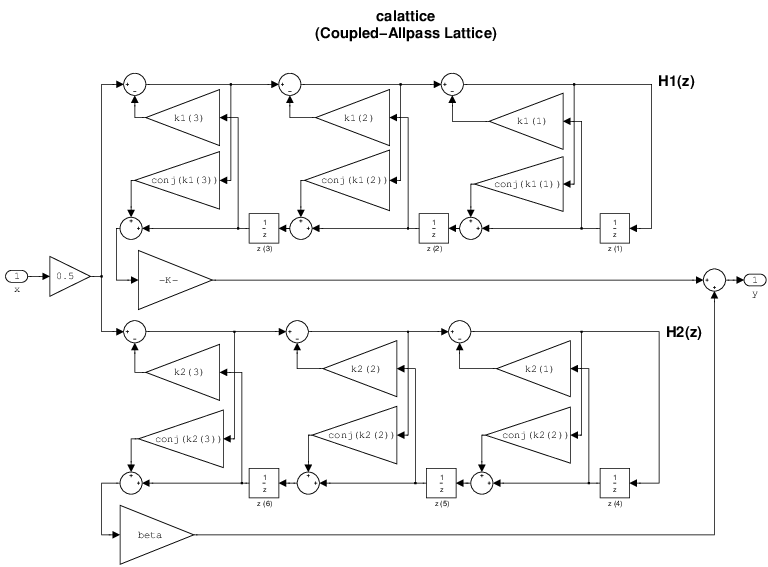Documentation

# dfilt.calattice

Coupled-allpass, lattice filter

## Syntax

```hd = dfilt.calattice(k1,k2,beta) hd = dfilt.calattice ```

## Description

`hd = dfilt.calattice(k1,k2,beta)` returns a discrete-time, coupled-allpass, lattice filter object `hd`, which is two allpass, lattice filter structures coupled together. The lattice coefficients for each structure are vectors `k1` and `k2`. Input argument `beta` is shown in the diagram below.

`hd = dfilt.calattice` returns a default, discrete-time coupled-allpass, lattice filter object, `hd`. The default values are `k1` = `k2` = [], and `beta` = 1. This filter passes the input through to the output unchanged.## Examples

Specify a third-order lattice coupled-allpass filter structure for a `dfilt` filter, `hd` with the following code.

```k1 = [0.9511 + 1j*0.3088; 0.7511 + 1j*0.1158]; k2 = 0.7502 - 1j*0.1218; beta = 0.1385 + 1j*0.9904; hd = dfilt.calattice(k1,k2,beta);```

The `Allpass1` and `Allpass2` properties store vectors of coefficients.

Watch now